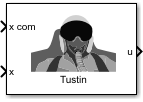# Tustin Pilot Model

Represent Tustin pilot model

• Library:
• Aerospace Blockset / Pilot Models

•## Description

The Tustin Pilot Model block represents the pilot model that A. Tustin describes in The Nature of the Operator’s Response in Manual Control, and its Implications for Controller Design . When modeling human pilot models, use this block for the least accuracy, compared to that provided by the Crossover Pilot Model and Precision Pilot Model blocks. This block requires less input than those blocks, and provides better performance. However, the results might be less accurate.

This pilot model is a single input, single output (SISO) model that represents human behavior, and is based on the transfer function described in Algorithms.

This block has nonlinear behavior. If you want to linearize the block (for example, with one of the `linmod` functions), you might need to change the Pade approximation order. The Tustin Pilot Model block implementation incorporates the Transport Delay block with the Pade order (for linearization) parameter set to `2` by default. To change this value, use the `set_param` function, for example:

`set_param(gcb,'pade','3')`

## Ports

### Input

expand all

Signal command that the pilot model controls, specified as a scalar.

Data Types: `double`

Signal controlled by pilot, specified as a scalar.

Data Types: `double`

### Output

expand all

Aircraft command, returned as a scalar.

Data Types: `double`

## Parameters

expand all

Pilot gain, specified as a double scalar.

#### Programmatic Use

 Block Parameter: `Kp` Type: character vector Values: double scalar Default: `'1'`

Total pilot time delay, specified as a double scalar, in seconds. This value typically ranges from 0.1 s to 0.2 s.

#### Programmatic Use

 Block Parameter: `time_delay` Type: character vector Values: double scalar Default: `'0.1'`

Pilot lead constant, specified as a double scalar.

#### Programmatic Use

 Block Parameter: `T` Type: character vector Values: double scalar Default: `'5'`

## Algorithms

This pilot model is a single input, single output (SISO) model that represents human behavior, based on the transfer function:

`$\frac{u\left(s\right)}{e\left(s\right)}=\frac{{K}_{p}\left(1+Ts\right)}{s}{e}^{-\tau s}.$`

In this equation:

VariableDescription
K p Pilot gain.